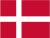### Dish Cloth 23

Cast on 60 stitches and knit 2 rows and then pattern. Remember to start and end all rows with 2 k.
Knit until the desired length ( three times pattern) and end with one row purl and  one row knit - bind off.

1.row : purl
2.row : knit
3.row : knit
4.row : 13 p, 1 k
5.row : 2 p, 11 k, 1 p
6.row : 1 p,1 k, 9 p, 1 k, 1 p, 1 k
7.row : 1 k, 3 p, 7 k, 3 p
8.row : 1 p, 1 k, 4 p, 1 k, 4 p, 1 k, 1 p, 1 k
9.row : 2 p, 4 k, 3 p, 4 k, 1 p
10.row : 4 p, 5 k, 4 p, 1 k
11.row : 4 k, 1 p, 1 k, 3 p, 1 k, 1 p, 3 k
12.row : 2 p, 3 k, 1 p, 1 k, 1 p, 3 k, 3 p
13.row : 2 k, 5 p, 1 k, 5 p, 1 k
14.row : as row 12
15.row : as row 11
16.row : as row 10
17.row : as row 9
18.row : as row 8
19.row : as row 7
20.row : as row 6
21.row : as row 5
22.row : as row 4
23.row : knit
24.row : knit
25.row : purl
26.row : purl

 26 X X X X X X X X X X X X X X 25 X X X X X X X X X X X X X X 24 23 X 22 X X X 21 X X X 20 X X X X X X 19 X X X X 18 X X X X X X 17 X X X X X X 16 X X X X X 15 X X X X X X X 14 X X X X X X X X X X 13 X X X X X X X 12 X X X X X 11 X X X X X X 10 X X X X X X 9 X X X X 8 X X X X X X 7 X X X 6 X X X 5 X 4 3 X X X X X X X X X X X X X X 2 X X X X X X X X X X X X X X 1
You have to remember that all uneven rows start in the right side of the diagram and X=purl and ▢= knit. All equal rows start in the left side of the diagram and X=knit and ▢=purl.Danish